# CNN（卷积神经网络） - 卷积计算提取图像边缘

webpro 神经网络算法 2018-09-11 643 0

CNN（卷积神经网络）算法一般应用在图像识别领域，如对数字字母的识别、区分猫狗等。卷积神经网络运算的第一步即使对输入图像进行边缘提<?php
/**
* 基于卷积神经网络算法的图像识别
* Bill
* 2018年9月7日 08点46分
*/
ini_set('memory_limit','-1');
set_time_limit(0);

// $imagePath = './ehuixue/1.jpg'; //$imagePath = './img/1.jpg';
// $imagePath = './img/crh.jpg';$imagePath = './img/train.jpg';

$array = getimagesize($imagePath);
print_r($array); echo '<br/>'; echo '<h2>【图像初始样式 - 60%】</h2>'; echo '<img width="60%" src="'.$imagePath.'"/>';
echo '<br/>';
echo '<br/>';
echo '<br/>';

$res = imagecreatefromjpeg($imagePath);
$size = getimagesize($imagePath);
$maxX =$size;
$maxY =$size;
// // echo "<pre>";
// // echo "<table border=\"1\" cellspacing=\"0\" cellpadding=\"0\">";
// // for ($i = 0;$i < $size; ++$i) { // 行
// //     echo "<tr>";
// //     for ($j = 0;$j < $size; ++$j) { // 列
// //         $rgb = imagecolorat($res, $j,$i);
// //         $rgbarray = imagecolorsforindex($res, $rgb); // // echo "<td>"; // // echo$rgbarray['red'].',<br/>'.$rgbarray['green'].',<br/>'.$rgbarray['blue'];
// //         echo "</td>";
// //     }
// //     echo "<tr>";
// // }
// // echo "</table>";
// // echo "</pre>";
// // echo "<br/>";
$input = array();$emmm = imagecreatetruecolor($size,$size);
for ($i = 0;$i < $size; ++$i) { // x
for ($j = 0;$j < $size; ++$j) { // y
$rgb = imagecolorat($res, $i,$j);
$rgbarray = imagecolorsforindex($res, $rgb);$rgb = (int)(($rgbarray['red'] +$rgbarray['green']/3 + $rgbarray['blue'])/12); if($rgb > 20){
$color = imagecolorallocate($emmm, 255, 255, 255);
$input[$j][$i] = 255; }else{$color = imagecolorallocate(  $emmm, 0, 0, 0);$input[$j][$i] = 0;
}
// $color = imagecolorallocate($emmm, $rgb,$rgb, $rgb); //$color  =  imagecolorallocate ( $emmm , 255 , 0 , 0 );// 设置颜色 - ps里的选择画笔颜色 imagesetpixel ($emmm , $i ,$j , $color ); } } date_default_timezone_set('PRC');$now = time();
echo '<h2>【灰度化 - 60%】</h2>';
ImagePNG($emmm,'./img/'.$now.'.png');
// imagepng($emmm); imagedestroy($emmm);// 销毁图片，释放内存
echo '<img width="60%" src="./img/'.$now.'.png"/>'; echo '<br/>'; //$red  =  imagecolorallocate ( $image , 255 , 0 , 0 );// 设置颜色 - ps里的选择画笔颜色 // for($i=0; $i<300; ++$i){
//     imagesetpixel (  $emmm , 100 ,$i , $red ); // } // header('Content-Type:image/jpg'); ///////////////////////////////////////////////////////////// ///////////////////////////////////////////////////////////// ///////////////////////////////////////////////////////////// // 二值化// 二值化// 二值化// 二值化// 二值化// 二值化// 二值化// // 二值化// 二值化// 二值化// 二值化// 二值化// 二值化// 二值化// // date_default_timezone_set('PRC'); //$now = time();
// ImagePNG($emmm,'./img/'.$now.'.png');
// // imagepng($emmm); // imagedestroy($emmm);// 销毁图片，释放内存
// echo '<img src="./img/'.$now.'.png"/>'; // echo '<br/>'; // foreach($input as $key =>$value){ // 先固定行，即y坐标，$j是y // foreach($value as $inner =>$x){
//         if($x < 100){ // echo '1'; // }else{ // echo '0'; // } // } // echo '<br/>'; // } ///////////////////////////////////////////////////////////// ///////////////////////////////////////////////////////////// ///////////////////////////////////////////////////////////// // 二值化// 二值化// 二值化// 二值化// 二值化// 二值化// 二值化// // 二值化// 二值化// 二值化// 二值化// 二值化// 二值化// 二值化// // print_r($input);

// 暂时不需要

// $input =$temp;
// print_r($input); // exit; // for ($i = 0; $i <$x; ++$i ) { // 列 // for ($j = 0; $j <$y; ++$j ) { // 行 // if ($input[$i][$j] < 80) {
//             echo "1";
//         }else{
//             echo "0";
//         }
//     }
//     echo '<br/>';
// }

$output = array(); // 卷积计算后输出 // 卷积计算 echo '<h2>【水平卷积过滤】</h2>'; /** X过滤，算子: 1 0 -1 1 0 -1 1 0 -1 */$wx = array(
0 => array( 1, 0, -1),
1 => array( 1, 0, -1),
2 => array( 1, 0, -1),
);
$wx = array( 0 => array( 1, -1, 1), 1 => array( 1, -1, 0), 2 => array( 1, -1, -1), ); for($i = 0; $i < ($maxY - 2); ++$i ){ // 固定行 for($j = 0; $j < ($maxX - 2); ++$j ){ // 遍历列$output[$i+1][$j+1] = 0; // 赋初始值
$temp = array( 0 => array($input[$i+0][$j+0], $input[$i+1][$j+0],$input[$i+2][$j+0] ),
1 => array( $input[$i+0][$j+1],$input[$i+1][$j+1], $input[$i+2][$j+1] ), 2 => array($input[$i+0][$j+2], $input[$i+1][$j+2],$input[$i+2][$j+2] ),
);
// 卷积计算
for( $m = 0;$m < 3; ++$m){ for($n = 0; $n < 3; ++$n){
$output[$i+1][$j+1] +=$temp[$m][$n] * $wx[$m][$n]; } } } } echo "<br/>"; echo "<table border=\"1\" cellspacing=\"0\" cellpadding=\"0\">"; for($i = 0; $i < ($maxY - 2); ++$i ){ // 固定行 echo "<tr>"; for($j = 0; $j < ($maxX - 2); ++$j ){ // 遍历列 echo "<td>"; echo reluActive($output[$i+1][$j+1]);
echo "</td>";
}
echo "</tr>";
}
echo "</table>";

echo "<br/>";
$emmm = imagecreatetruecolor($maxX - 2, $maxY - 2); for($i = 0; $i < ($maxY - 2); ++$i ){ // 固定行 echo "<tr>"; for($j = 0; $j < ($maxX - 2); ++$j ){ // 遍历列$rgb = $output[$i+1][$j+1];$color = imagecolorallocate(  $emmm,$rgb, $rgb,$rgb);
imagesetpixel (  $emmm ,$j , $i ,$color );
}
}
date_default_timezone_set('PRC');
$now = time(); ImagePNG($emmm,'./img/filtered'.$now.'.png'); // imagepng($emmm);
imagedestroy($emmm);// 销毁图片，释放内存 echo '<img width="60%" src="./img/filtered'.$now.'.png"/>';
echo '<br/>';

$output1 = array(); // 卷积计算后输出 echo '<h2>【垂直卷积过滤】</h2>'; /** Y过滤，算子: 1 1 1 0 0 0 -1 -1 -1 */$wy = array(
0 => array( 1, 1, 1),
1 => array( 0, 0, 0),
2 => array( -1, -1, -1),
);

for( $i = 0;$i < ($maxY - 2); ++$i ){ // 固定行
for( $j = 0;$j < ($maxX - 2); ++$j ){ // 遍历列
$output1[$i+1][$j+1] = 0; // 赋初始值$temp = array(
0 => array( $input[$i+0][$j+0],$input[$i+1][$j+0], $input[$i+2][$j+0] ), 1 => array($input[$i+0][$j+1], $input[$i+1][$j+1],$input[$i+2][$j+1] ),
2 => array( $input[$i+0][$j+2],$input[$i+1][$j+2], $input[$i+2][$j+2] ), ); // 卷积计算 for($m = 0; $m < 3; ++$m){
for( $n = 0;$n < 3; ++$n){$output1[$i+1][$j+1] += $temp[$m][$n] *$wy[$m][$n];
}
}
}
}

echo "<br/>";

for( $i = 0;$i < ($maxY - 2); ++$i ){ // 固定行
echo "<tr>";
for( $j = 0;$j < ($maxX - 2); ++$j ){ // 遍历列
echo "<td>";
echo reluActive($output1[$i+1][$j+1]); echo "</td>"; } echo "</tr>"; } echo "</table>"; echo "<br/>";$emmm = imagecreatetruecolor($maxX - 2,$maxY - 2);
for( $i = 0;$i < ($maxY - 2); ++$i ){ // 固定行
echo "<tr>";
for( $j = 0;$j < ($maxX - 2); ++$j ){ // 遍历列
$rgb =$output1[$i+1][$j+1];
$color = imagecolorallocate($emmm, $rgb,$rgb, $rgb); imagesetpixel ($emmm , $j ,$i , $color ); } } date_default_timezone_set('PRC');$now = time();
ImagePNG($emmm,'./img/filtered1'.$now.'.png');
// imagepng($emmm); imagedestroy($emmm);// 销毁图片，释放内存
echo '<img width="60%" src="./img/filtered1'.$now.'.png"/>'; echo '<br/>';$final = array();

echo '<h2>【X+Y卷积结果】</h2>';

// XY合并
for( $i = 0;$i < ($maxY - 2); ++$i ){ // 固定行
for( $j = 0;$j < ($maxX - 2); ++$j ){ // 遍历列
$final[$i][$j] = ($output[$i+1][$j+1])?($output[$i+1][$j+1]):($output1[$i+1][$j+1]);
}
}
echo "<br/>";

for( $i = 0;$i < ($maxY - 2); ++$i ){ // 固定行
echo "<tr>";
for( $j = 0;$j < ($maxX - 2); ++$j ){ // 遍历列
echo "<td>";
echo reluActive($final[$i][$j]); echo "</td>"; } echo "</tr>"; } echo "</table>"; echo "<br/>";$emmm = imagecreatetruecolor($maxX - 2,$maxY - 2);
for( $i = 0;$i < ($maxY - 2); ++$i ){ // 固定行
echo "<tr>";
for( $j = 0;$j < ($maxX - 2); ++$j ){ // 遍历列
$rgb =$final[$i][$j];
$color = imagecolorallocate($emmm, $rgb,$rgb, $rgb); imagesetpixel ($emmm , $j ,$i , $color ); } } date_default_timezone_set('PRC');$now = time();
ImagePNG($emmm,'./img/final'.$now.'.png');
// imagepng($emmm); imagedestroy($emmm);// 销毁图片，释放内存
echo '<img width="60%" src="./img/final'.$now.'.png"/>'; echo '<br/>'; function reluActive($x){
return max(0, \$x);
}=================================================

### 1.边界检测示例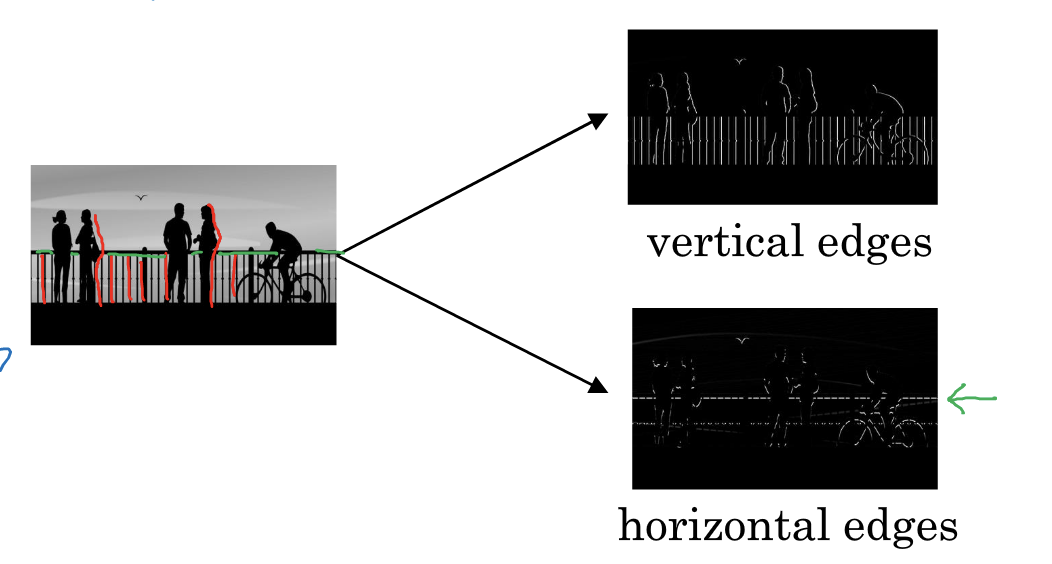3*1+0*0+1*-1+1*1+5*0+8*-1+2*1+7*0+2*-1 = -5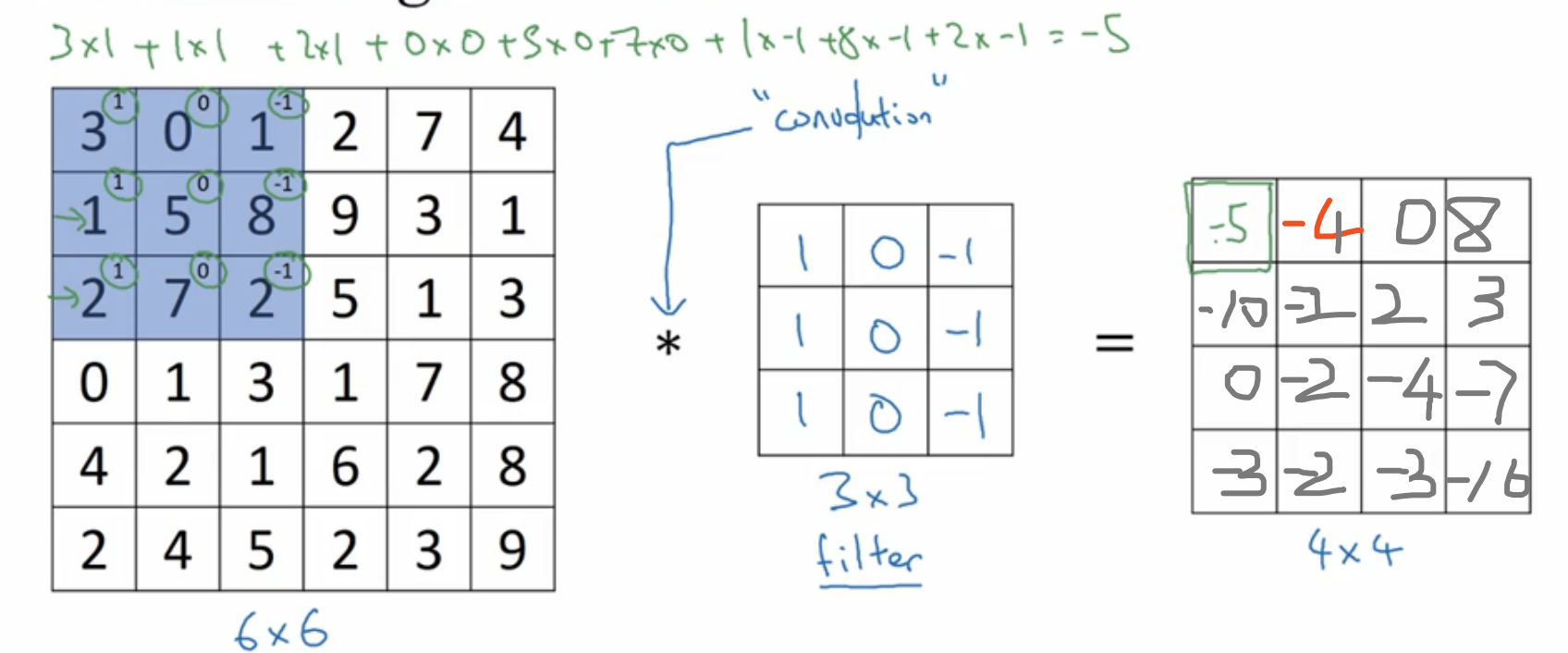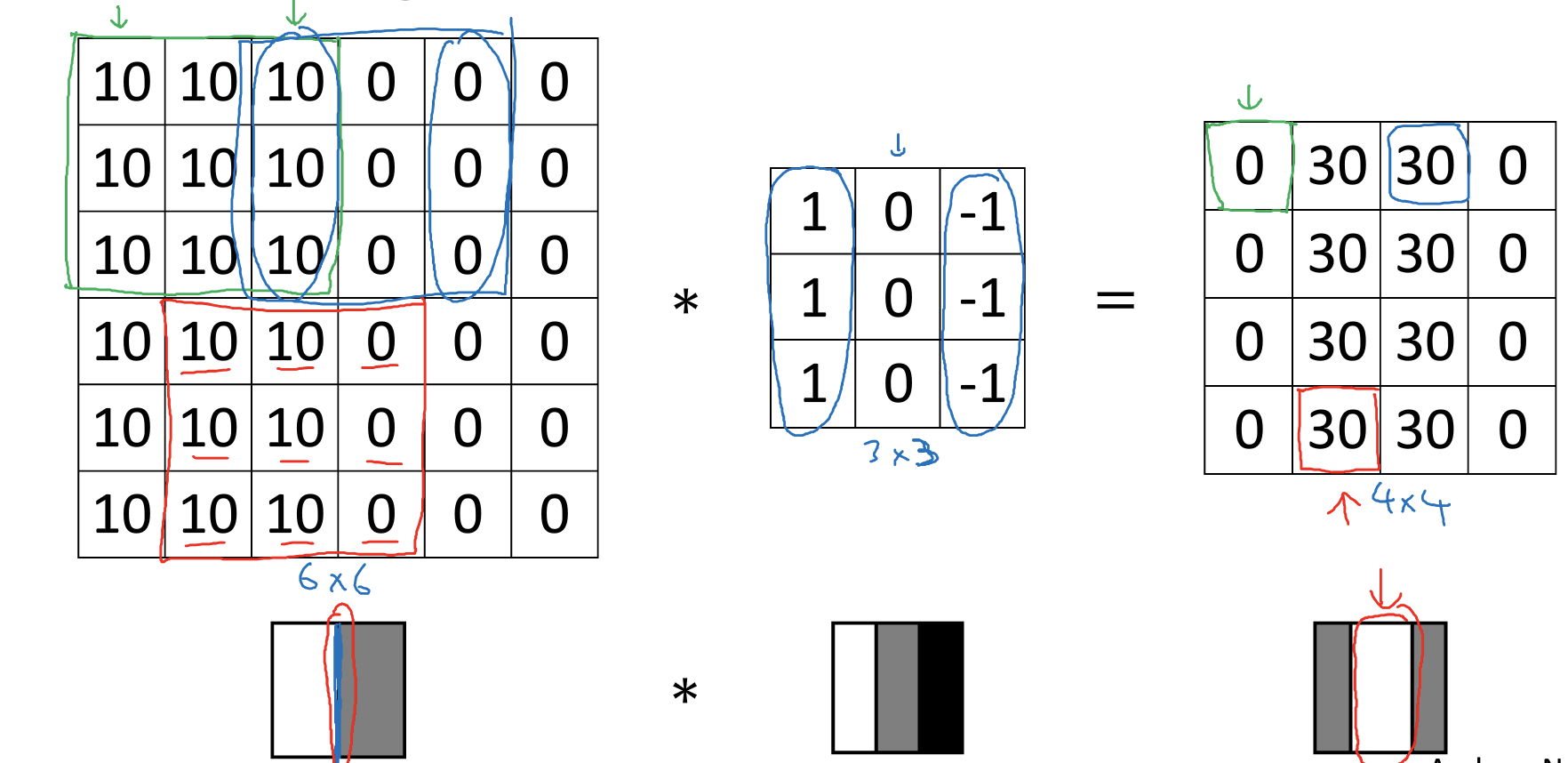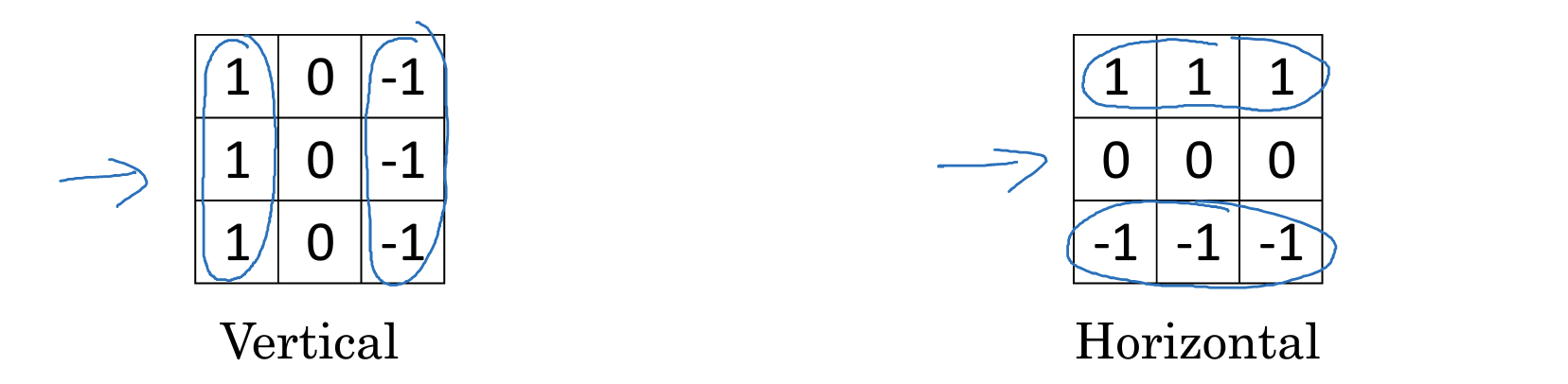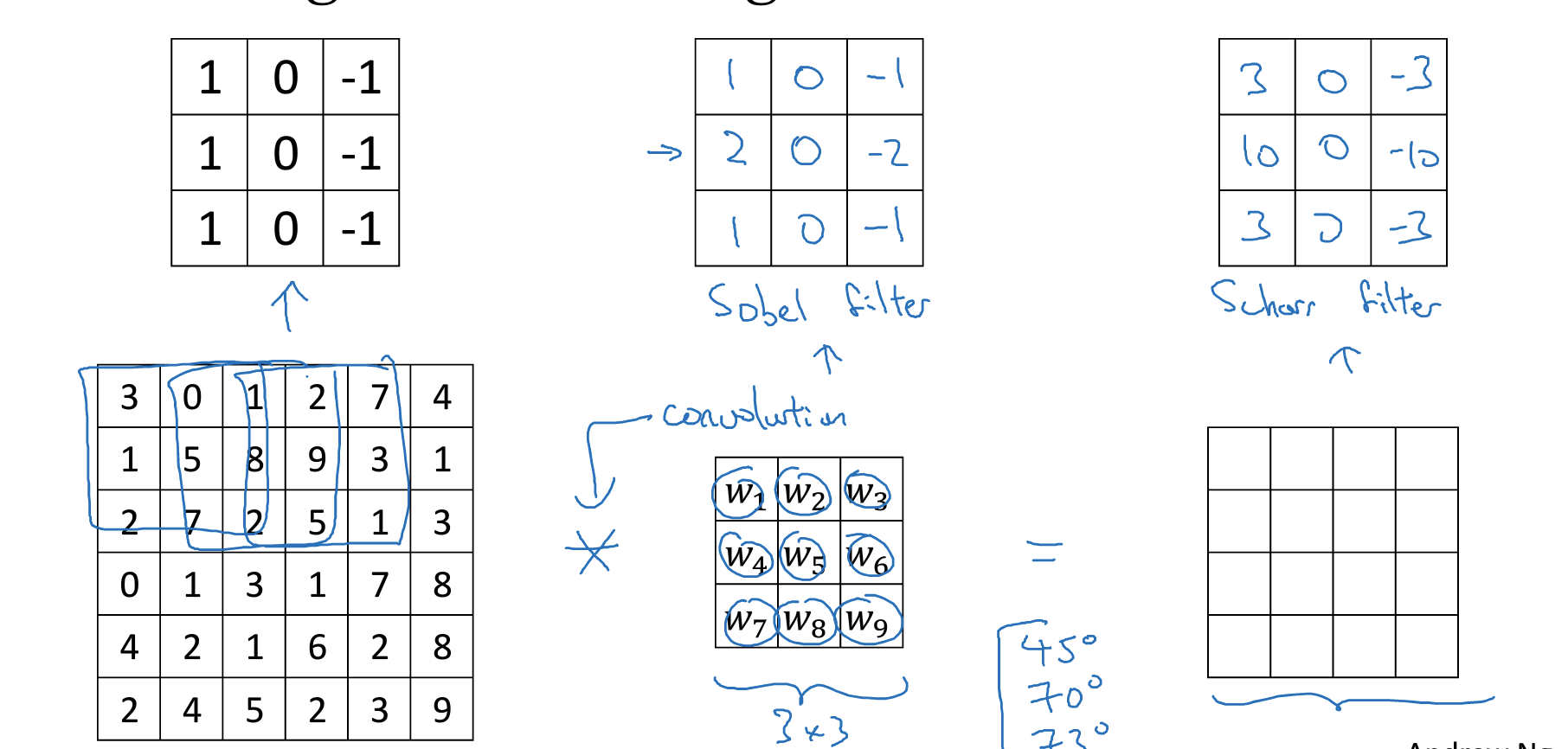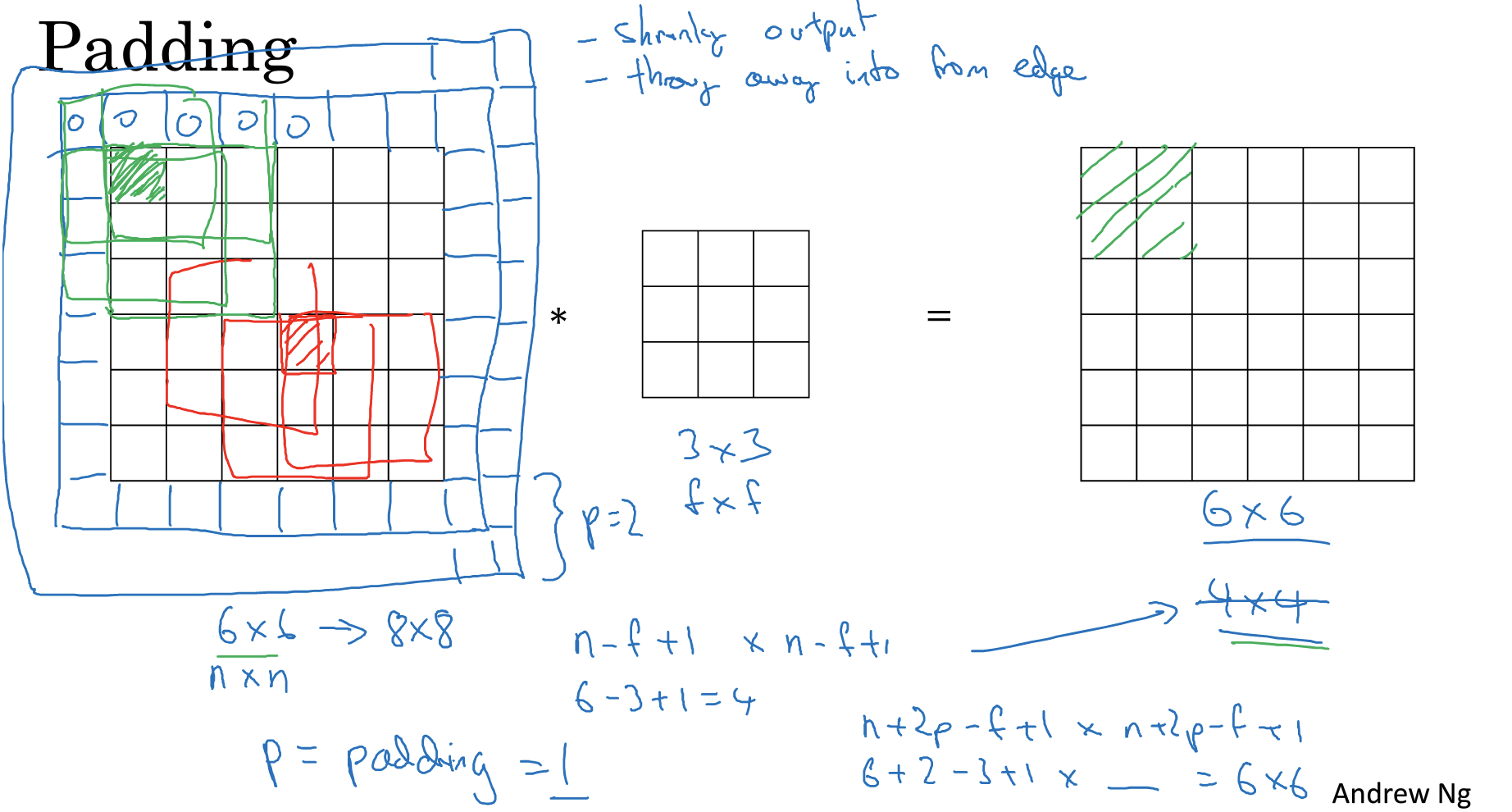Same：输出图像和输入图像一样大

### 3.卷积步长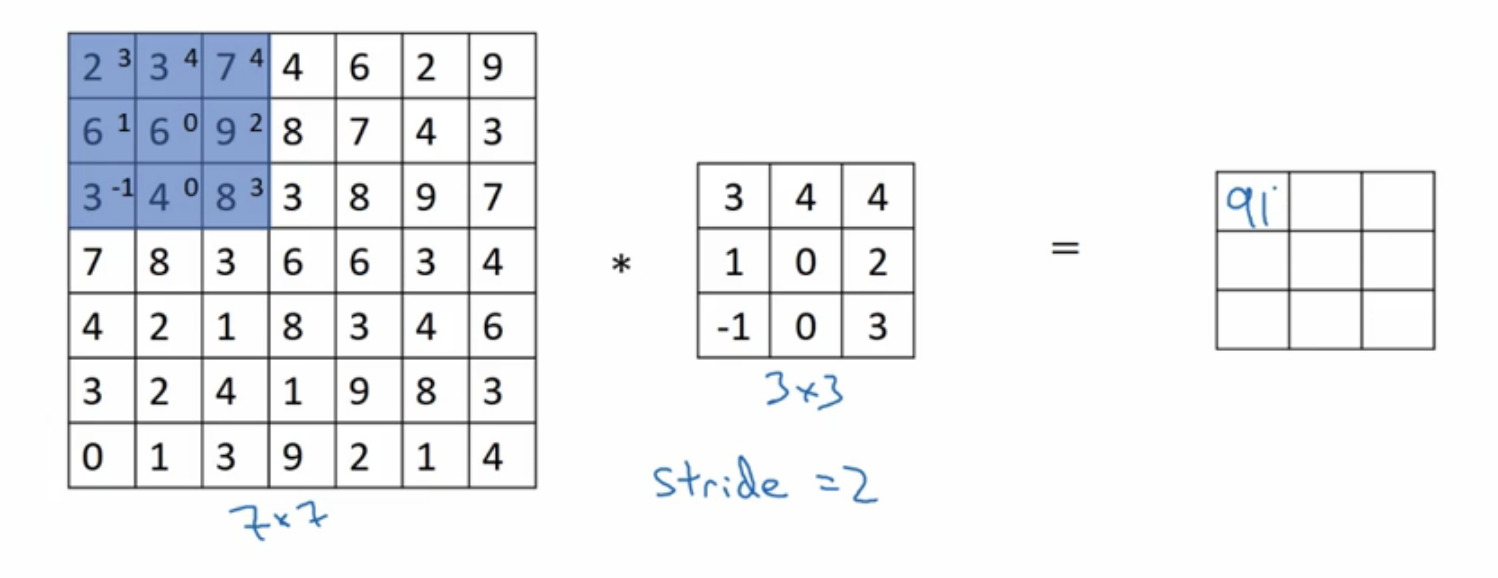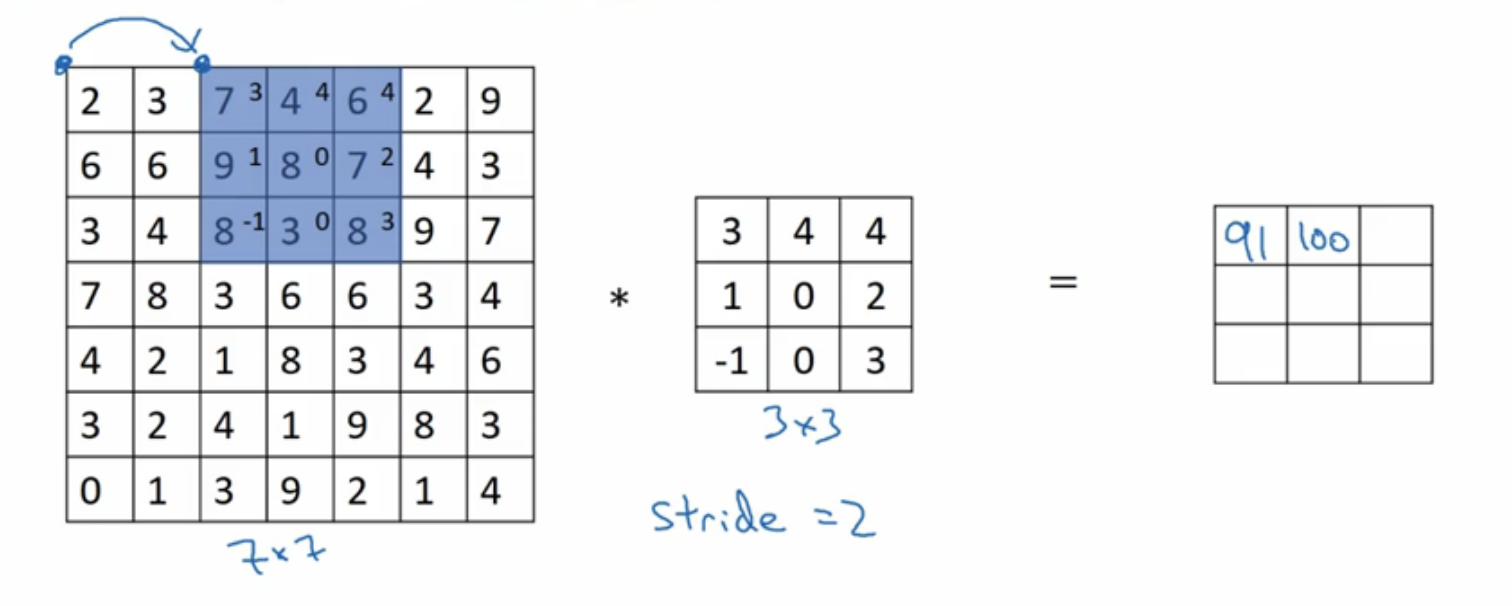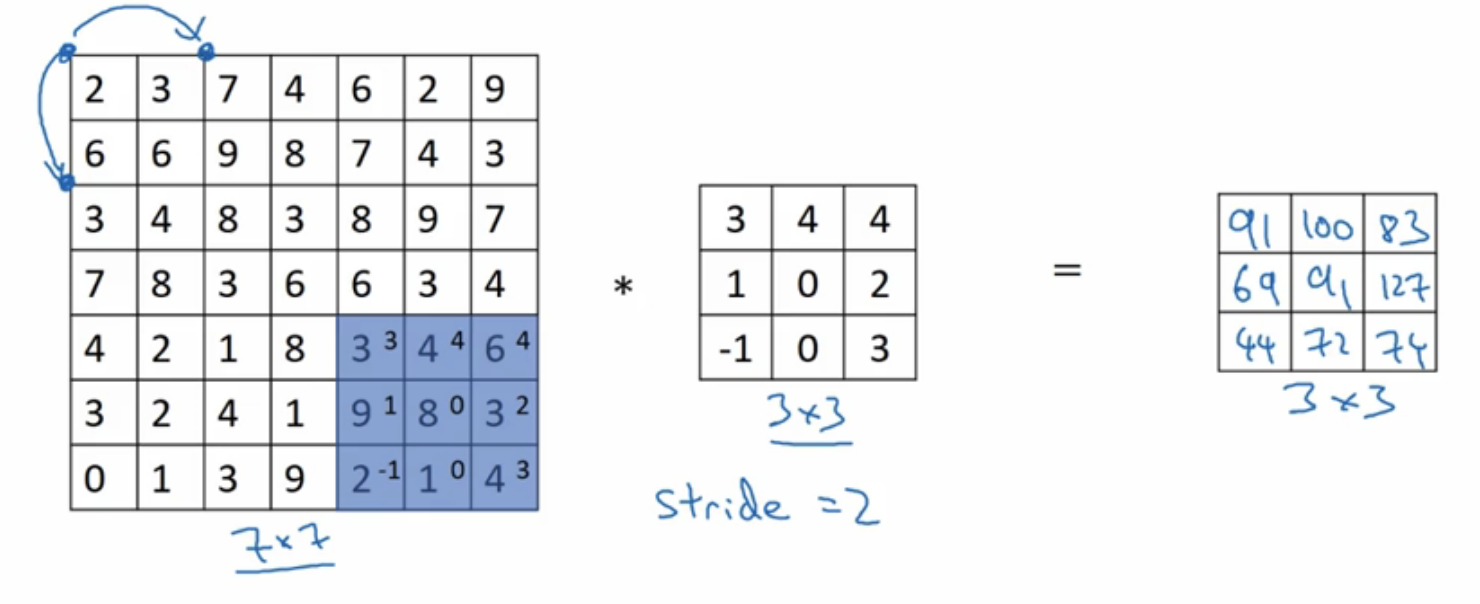### 4.彩色图像的卷积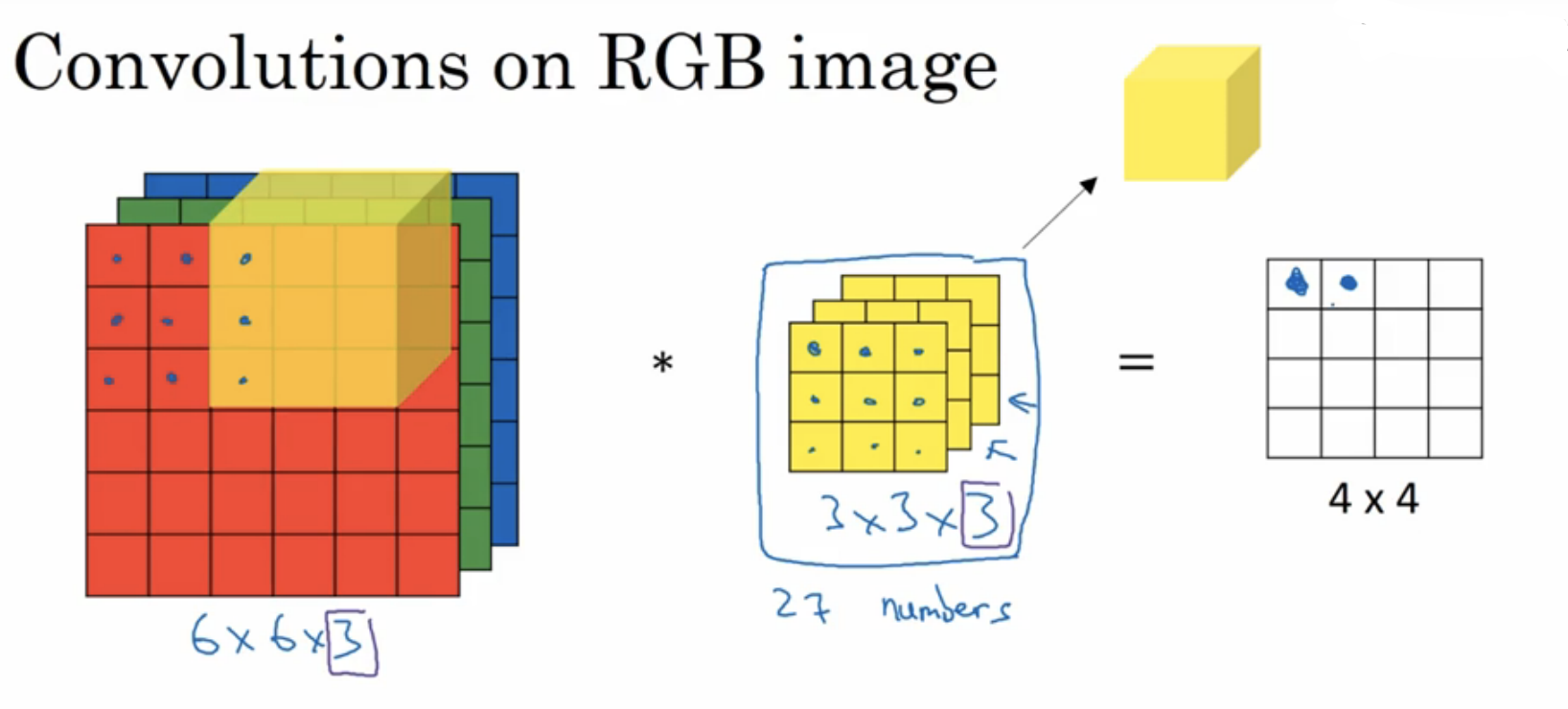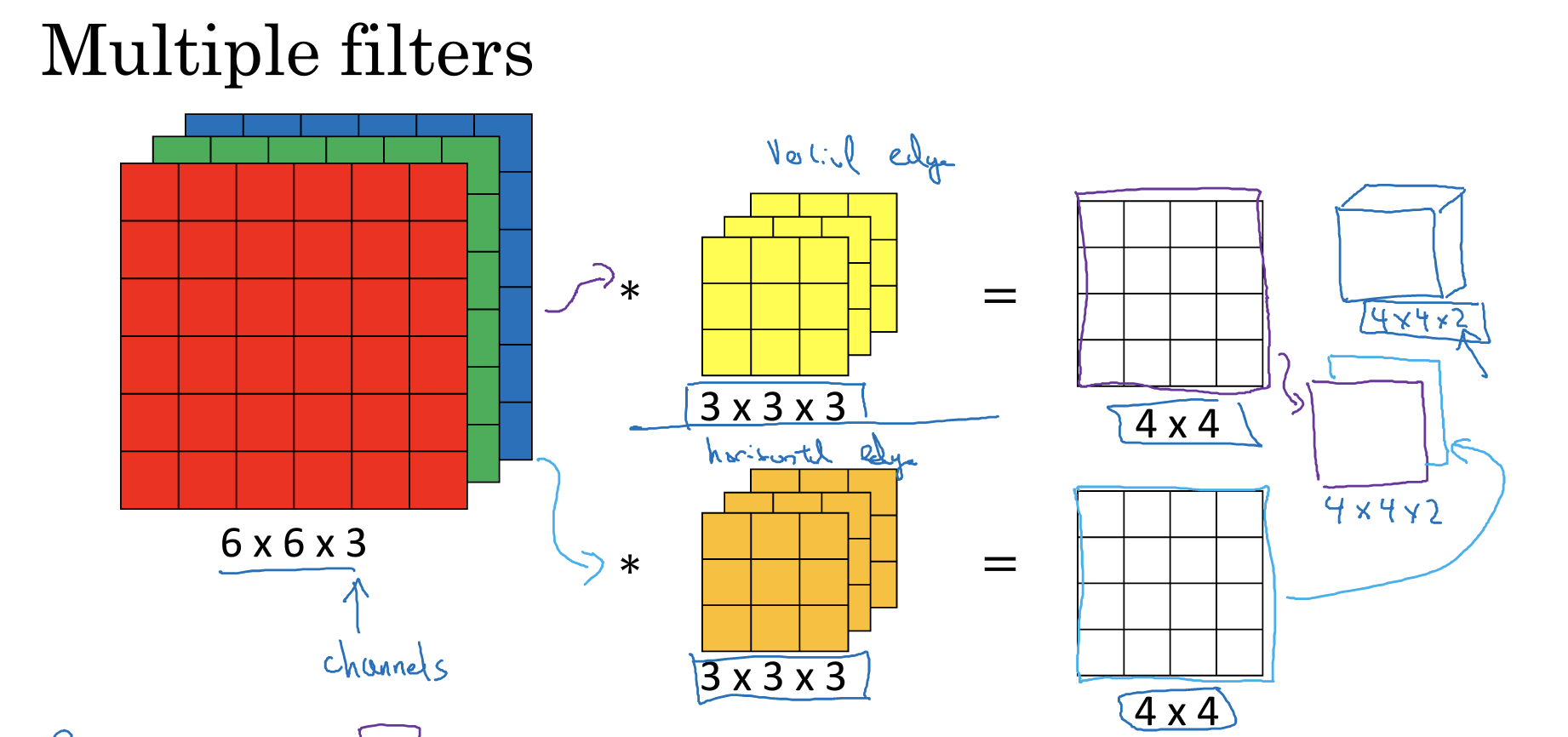### 5.单层卷积网络

${z}^{\left[1\right]}={w}^{\left[1\right]}{a}^{\left[0\right]}+{b}^{\left[1\right]}$
${a}^{\left[1\right]}=g\left({z}^{\left[1\right]}\right)$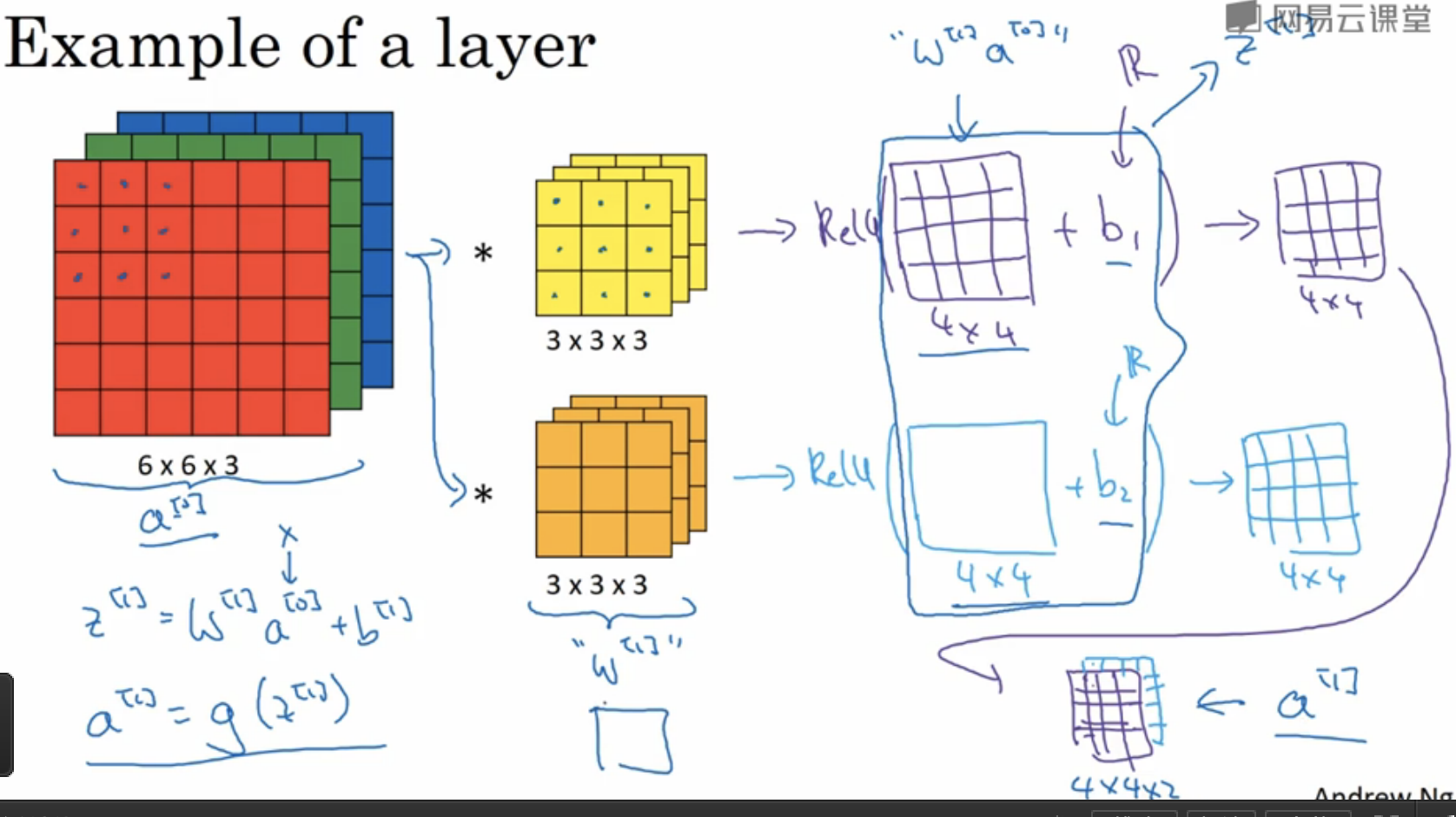• 如果有10个过滤器参数个数有多少个呢？
每个过滤器都有3*3*3+1=28个参数，3*3*3为过滤器大小，1是偏差系数，10个过滤器参数个数就是28*10=280个。不论输入图像大小参数个数是不会发生改变的。

• 描述卷积神经网络的一些符号标识：
$l$为一个卷积层：
${f}^{\left[l\right]}$：第$l$层过滤器的大小
${p}^{\left[l\right]}$：第$l$层padding的数量
${s}^{\left[l\right]}$：第$l$层步长大小
${n}_{C}^{l}$：过滤器的个数
Input:
${n}_{H}^{l-1}×{n}_{W}^{l-1}×{n}_{C}^{l-1}$$l-1$层输入图像的高、宽以及通道数。
Output：
${n}_{H}^{l}×{n}_{W}^{l}×{n}_{C}^{l}$：输出图像的高、宽以及通道数
输出图像的大小：
${n}_{H}^{l}=⌊\frac{{n}_{H}^{l-1}+2\ast {p}^{l}-{f}^{\left[l\right]}}{{s}^{l}}+1⌋$
${n}_{W}^{l}=⌊\frac{{n}_{W}^{l-1}+2\ast {p}^{l}-{f}^{\left[l\right]}}{{s}^{l}}+1⌋$
输出图像的通道数就是过滤器的个数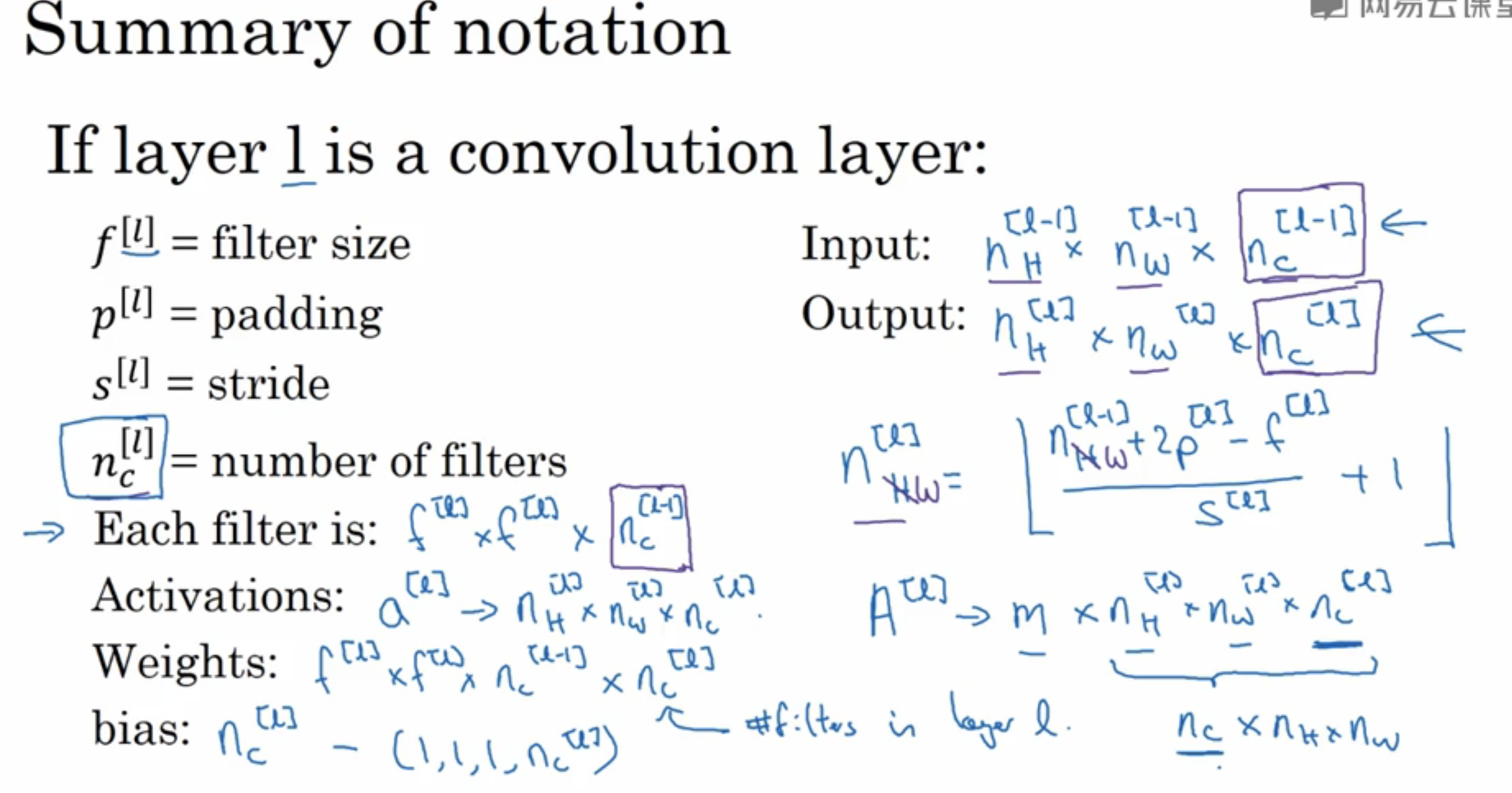### 6.简单卷积网络示例

• 输入图像：39*39*3，符号表示：${n}_{H}^{\left[0\right]}={n}_{W}^{\left[0\right]}=39$ $;{n}_{c}^{\left[0\right]}=3$

• 第1层超参数：${f}^{\left[l\right]}=3$(过滤器大小)；${s}^{\left[l\right]}=1$(步长)；${p}^{\left[l\right]}=0$(padding大小)；${n}_{C}^{\left[l\right]}=10$(过滤器个数)

• 第1层输出图像：37*37*10，符号表示：${n}_{H}^{\left[1\right]}={n}_{W}^{\left[1\right]}=37$ $;{n}_{c}^{\left[1\right]}=10$

• 第2层超参数：${f}^{\left[2\right]}=5$${s}^{\left[2\right]}=2$${p}^{\left[2\right]}=0$${n}_{C}^{\left[2\right]}=20$

• 第2层输出图像：17*17*20，符号表示：${n}_{H}^{\left[2\right]}={n}_{W}^{\left[2\right]}=17$ $;{n}_{c}^{\left[2\right]}=20$

• 第3层超参数：${f}^{\left[3\right]}=5$${s}^{\left[3\right]}=2$${p}^{\left[2\right]}=0$${n}_{C}^{\left[3\right]}=40$

• 第3层输出图像：7*7*40，符号表示：${n}_{H}^{\left[3\right]}={n}_{W}^{\left[3\right]}=17$ $;{n}_{c}^{\left[3\right]}=40$

• 将第三层的输出展开成1960个元素

• 然后将其输出到logistic或softmax来决定是判断图片中有没有猫，还是想识别图像中K中不同的对象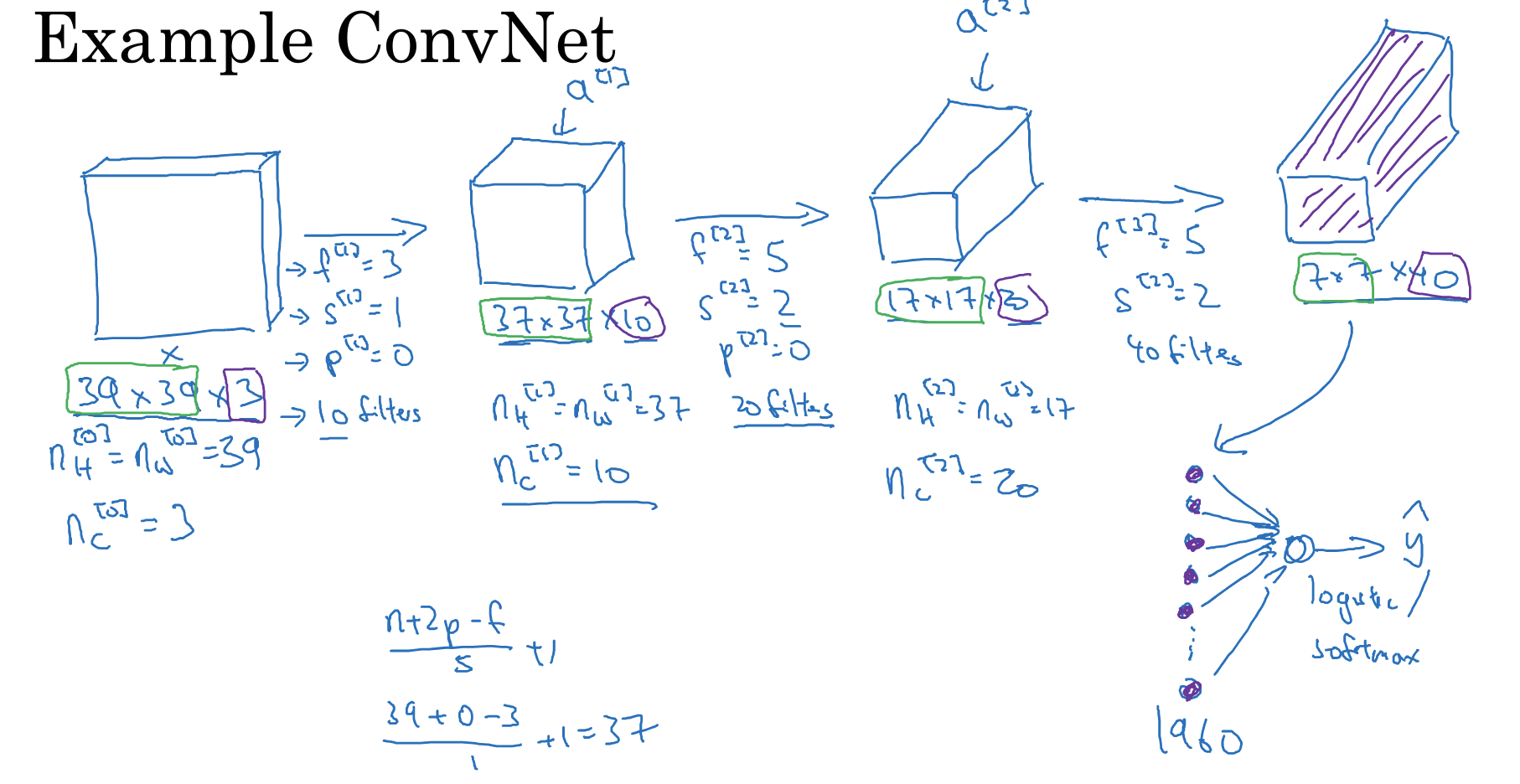卷积神经网络层的类型：

• 卷积层(convolution,conv)

• 池化层(pooling,pool)

• 全连接层(Fully connected,FC)

### 7.池化层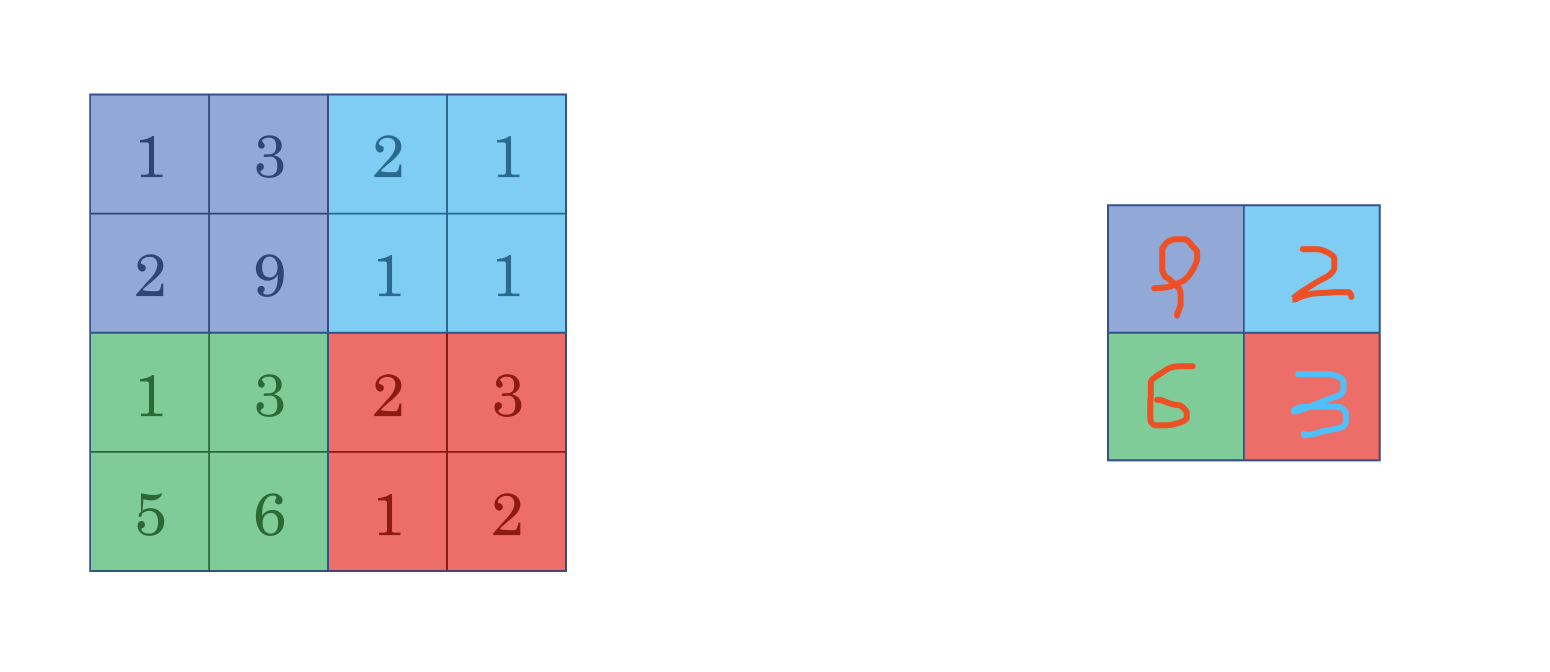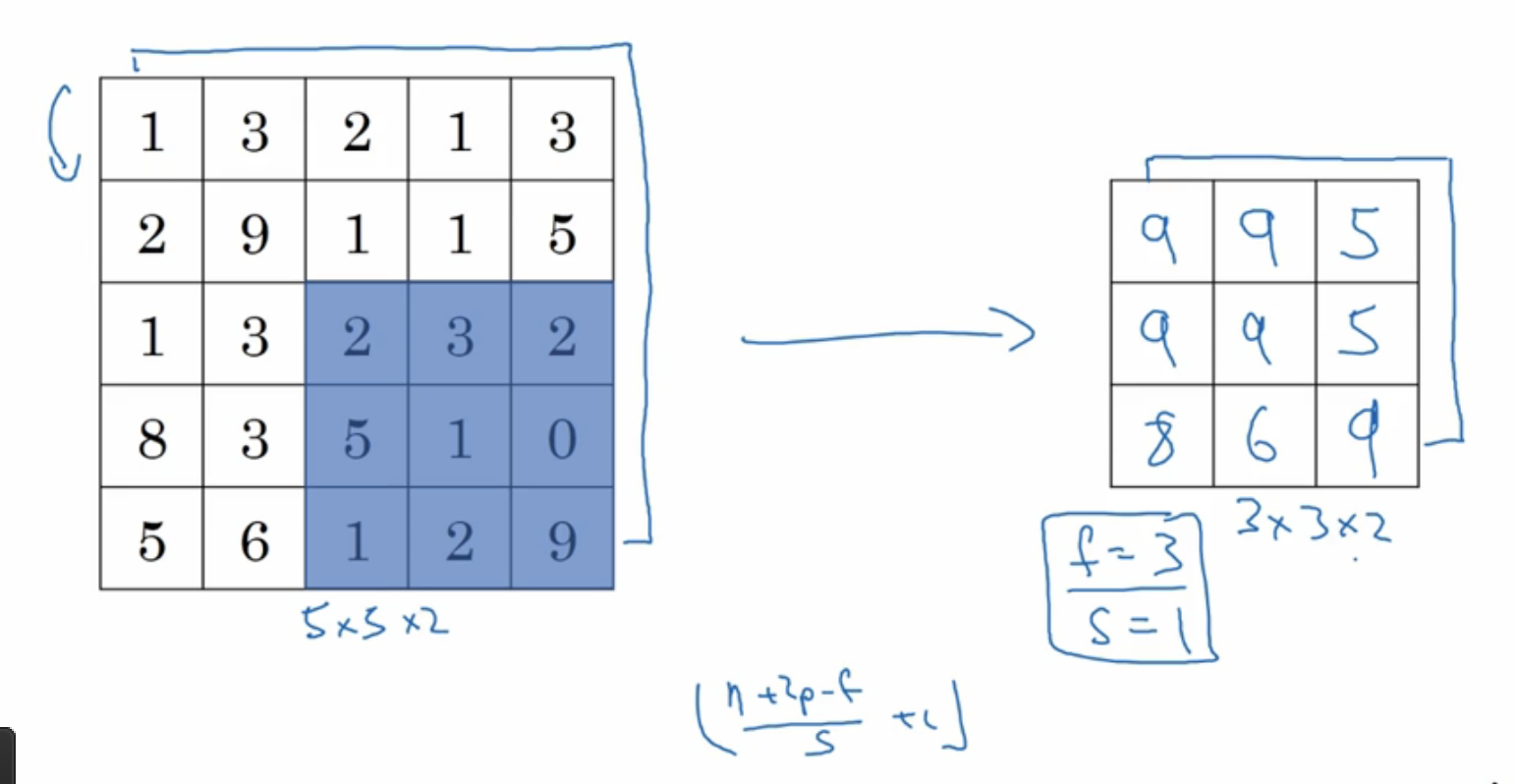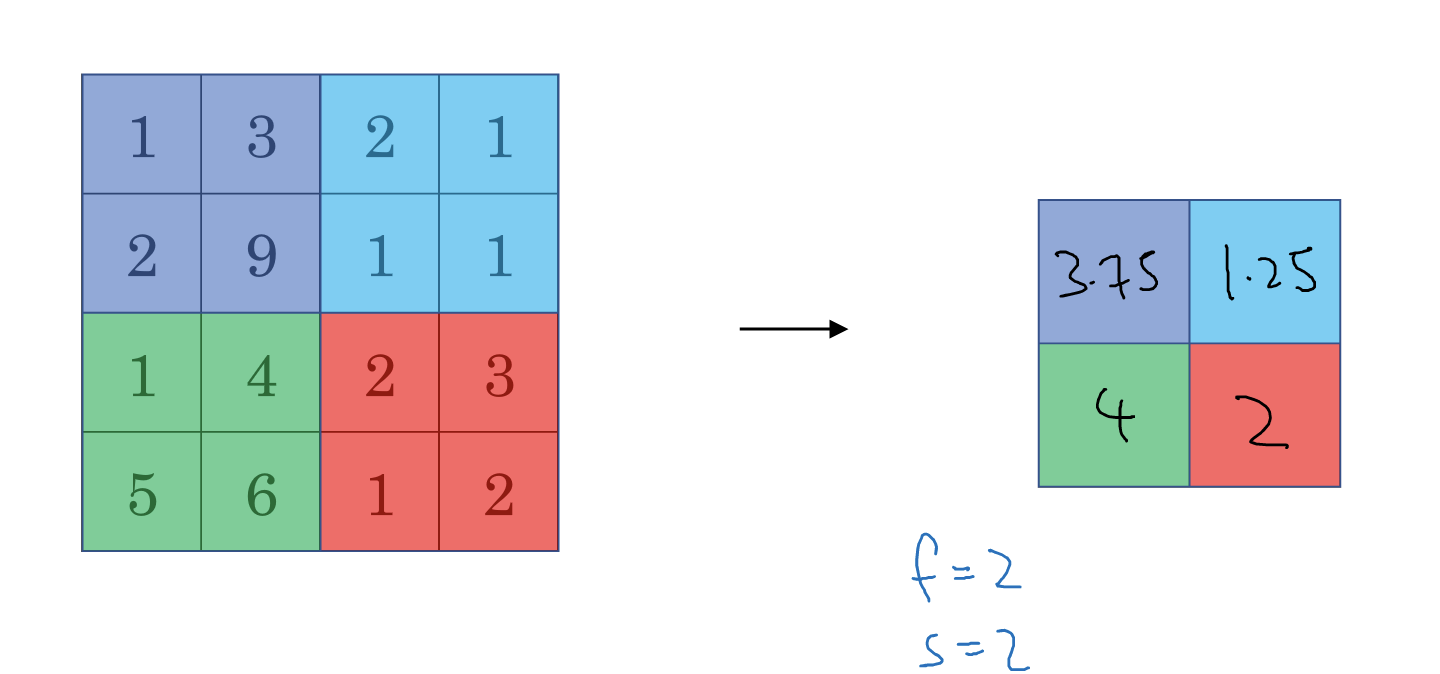### 8.卷积神经网络示例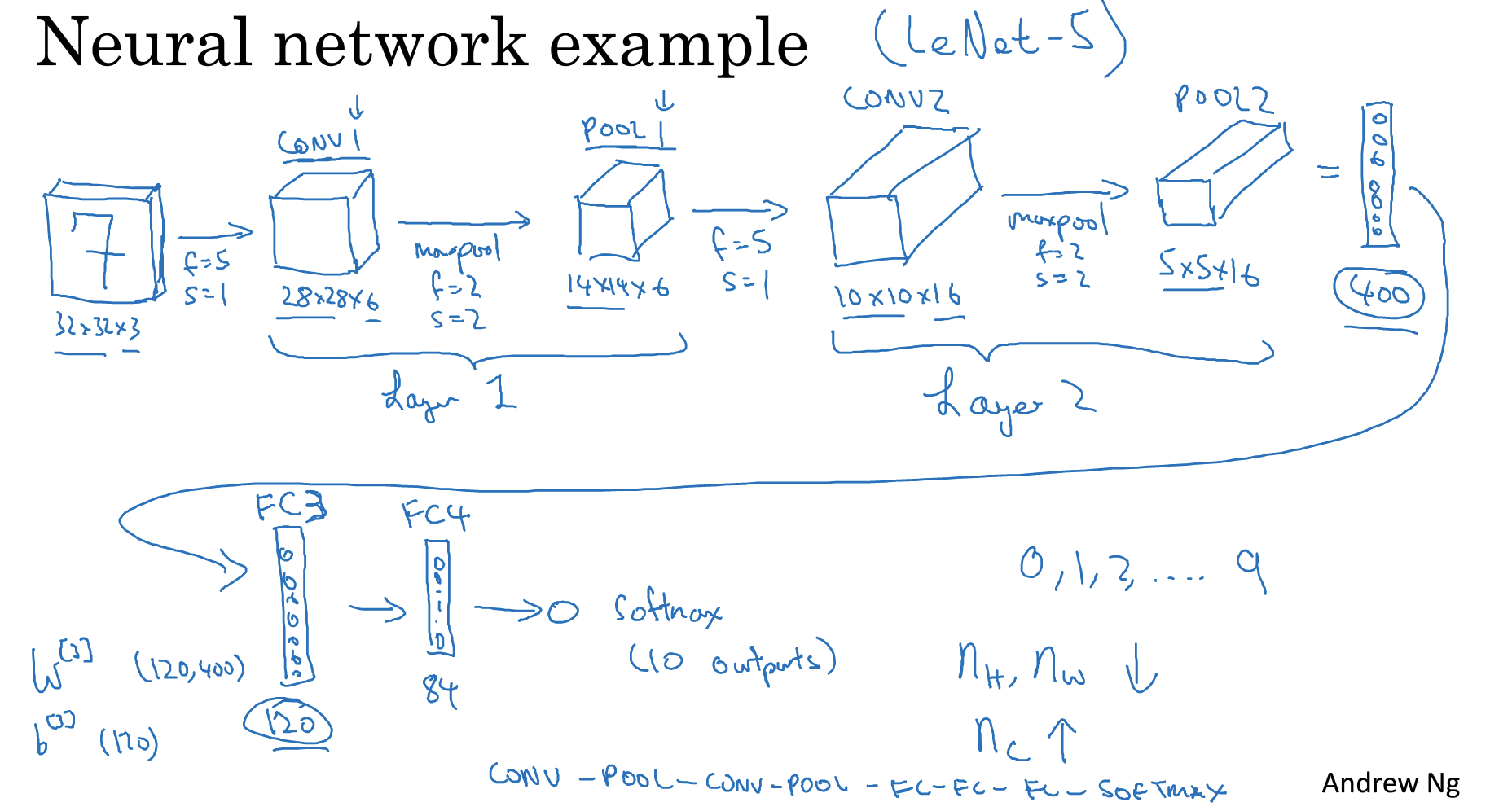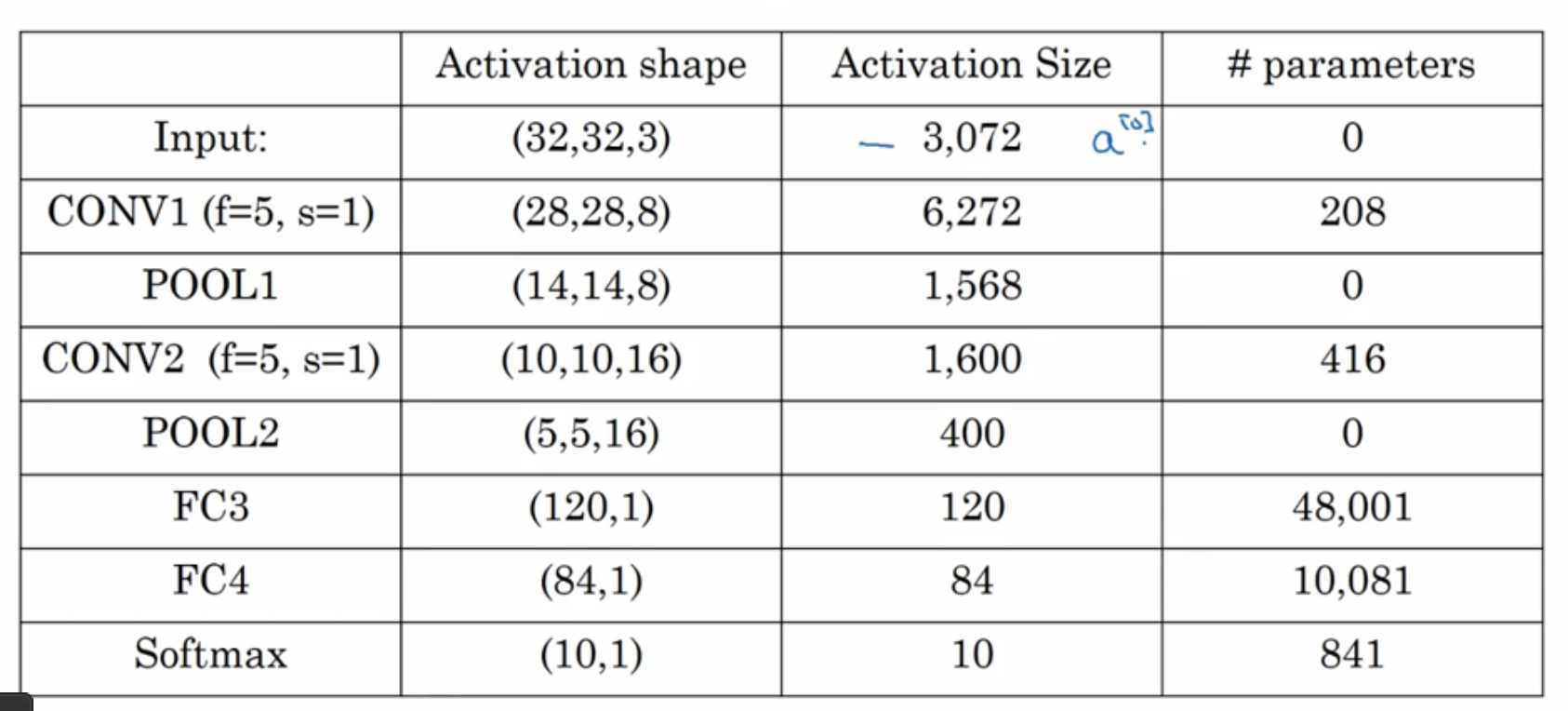Top